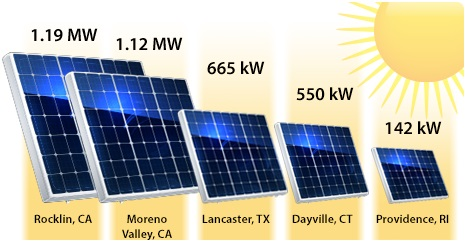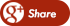﻿ Calculation for Size of a Solar PanelBack

## Calculation for Size of a Solar PanelCalculate Size of Solar Panel, No of Solar Panel and Size of Inverter for following Electrical Load

1 No’s of 100W Computer use for 8 Hours/Day

2 No’s of 60W Fan use for 8 Hours/Day

1 No’s of 100W CFL Light use for 8 Hours/Day

Solar System Detail:

Solar System Voltage (As per Battery Bank) = 48V DC

Loose Wiring Connection Factor = 20%

Daily Sunshine Hour in Summer = 6 Hours/Day

Daily Sunshine Hour in Winter = 4.5 Hours/Day

Daily Sunshine Hour in Monsoon = 4 Hours/Day

Inverter Detail:

Future Load Expansion Factor = 10%

Inverter Efficiency = 80%

Inverter Power Factor =0.8Calculation:

Step-1: Calculate Electrical  Usages per Day

Power Consumption for Computer = No x Watt x Use Hours/Day

Power Consumption for Computer = 1x100x8 =800  Watt Hr/Day

Power Consumption for Fan = No x Watt x Use Hours/Day

Power Consumption for Fan = 2x60x8 = 960  Watt Hr/Day

Power Consumption for CFL Light = No x Watt x Use Hours/Day

Power Consumption for CFL Light = 1x100x8 = 800 Watt Hr/Day

Total Electrical Load = 800+960+800 =2560 Watt  Hr/Day

Step-2: Calculate Solar  Panel Size

Average Sunshine Hours = Daily Sunshine Hour in Summer+ Winter+ Monsoon /3

Average Sunshine Hours = 6+4.5+4 / 3 =8 Hours

Total Electrical Load =2560 Watt Hr/Day

Required Size of Solar Panel = (Electrical Load  / Avg. Sunshine) X Correction Factor

Required Size of Solar Panel =(2560 / 4.8) x 1.2 =  635.6 Watt

Required Size of Solar Panel = 635.6 Watt

Step-3: Calculate No of  Solar Panel / Array of Solar Panel

If we Use 250 Watt, 24V  Solar Panel in Series-Parallel Type Connection

In Series-Parallel Connection Both Capacity (watt) and Volt are increases

No of String of Solar Panel (Watt) = Size of  Solar Panel / Capacity of Each Panel

No of String of Solar Panel ( Watt) = 635.6 / 250 = 2.5 No’s Say 3 No’s

No of Solar Panel in Each String= Solar System  Volt / Each Solar Panel Volt

No of Solar Panel in Each String= 48/24 =2  No’s

Total No of Solar Panel = No of String of Solar Panel x No of Solar Panel in Each String

Total No of Solar Panel = 3×2 =6 No’s

Total No of Solar Panel =6 No’s

Load for Computer = No x Watt

Load for Computer = 1×100 =100 Watt

Load for Fan = No x Watt

Load for Fan = 2×60 = 120 Watt

Load for CFL Light = No x Watt

Load for CFL Light = 1×100 = 100 Watt

Total Electrical Load = 100+120+100 =320 Watt

Step-5: Calculate Size  of Inverter:

Total Electrical Load in Watt = 320 Watt

Total Electrical Load in VA= Watt /P.F

Total Electrical Load in VA =320/0.8 = 400VA

Size of Inverter =Total Load x Correction Factor  / Efficiency

Size of Inverter = 320 x 1.2 / 80% =440  Watt

Size of Inverter =400 x 1.2 / 80% =600  VA

Size of Inverter = 440 Watt or 600 VASummary:

Required Size of Solar Panel = 635.6 Watt

Size of Each Solar Panel = 250 Watt. 12 V

No of String of Solar Panel = 3 No’s

No of Solar Panel in Each String = 2 No’s

Total No of Solar Panel =6 No’s

Total Size of Solar Panel = 750 Watt

Size of Inverter = 440 watt or 600 VA

FOR MORE DETAILS: CLICK HERE...